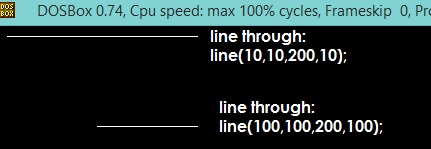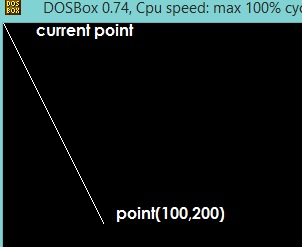# C graphics program to draw a line.

In this code snippet/program/example we will learn how to draw a line using programming using line() function of graphics.h header file?

In this example we will draw two horizontal lines using line() function of graphics.h.

#### line() in c programming:

line() is a library function of graphics.c in c programming language which is used to draw a line from two coordinates.

For example if you want to draw a line from point(x1,y1) to point(x2,y2) you have to use line() function like line(x1,y1,x2,y2);

Syntax (Declaration of line() function in C)

`line(int x1,int y1, int x2,int y2);`

## C Code Snippet - Graphics program to Draw a line using line() and lineto()

Let’s consider the following example:

```/*C graphics program to draw a line.*/

#include <graphics.h>
#include <conio.h>

main()
{
int gd = DETECT, gm;

//init graphics
initgraph(&gd, &gm, "C:/TURBOC3/BGI");
/*
if you are using turboc2 use below line to init graphics:
initgraph(&gd, &gm, "C:/TC/BGI");
*/

//draw a line

/*
line() function description
parameter left to right
x1: 100
y1: 100
x2: 200
y2: 100
*/
line(100,100,200,100);    //will draw a horizontal line

line(10,10,200,10); //will draw another horizonatl line

getch();
closegraph();
return 0;
}
```

### Output### Draw a line from current point to point(x,y) using lineto() function

lineto() is a library function of graphics.h and it can be used to draw a line from current point to specified point.

For example, if you want to draw a line from current point to point(x,y), you have to use lineto() function like lineto(x,y)

Syntax (Declaration of line() function in C)

`lineto(int x, int y);`

In this program we will draw a line from current point to point (100,200).

```/*Draw a line from current point to
point(x,y) using lineto() function*/

#include <graphics.h>
#include <conio.h>

main()
{
int gd = DETECT, gm;

//init graphics
initgraph(&gd, &gm, "C:/TURBOC3/BGI");
/*
if you are using turboc2 use below line to init graphics:
initgraph(&gd, &gm, "C:/TC/BGI");
*/

//draw a line
lineto(100,200); //will dra a line to point(100,200)

getch();
closegraph();
return 0;
}
```

#### OutputCompiler: TURBOC3, File extension: .c, Run under DOS BOX